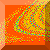# 2. Background

We refer to the collection of initial conditions whose orbits result in a particular behavior as the basin of that particular type of behavior. (This is a slight generalization of the concept of a basin of attraction to which it reduces if we identify the ``behaviors'' as motions on attractors.) A point p is a boundary point of a basin B if every open neighborhood of p intersects the basin B and at least one other basin. The set of all boundary points for a particular basin is the boundary of that basin. The basin boundary is termed fractal [Grebogi et al., 1983a, 1983b; Takesue & Kaneko, 1984; MacDonald et al., 1985; Moon & Li, 1985; Gwinn & Westervelt, 1986] when its box-counting dimension is not integral. In general, this implies the existence of an unstable chaotic invariant set embedded in the fractal basin boundary [Grebogi et al., 1986, 1987b, 1987c, 1988].

The term Wada property was used by Kennedy and Yorke  to describe sets which have certain interesting topological features. Specifically, a boundary point p is called a Wada point if every open neighborhood of p intersects at least three different basins. A basin boundary has this Wada property when every boundary point of the basin is a Wada point. The boundary for such a basin is called a Wada basin boundary.

The system we have elected to study to show this Wada property is a simple two-dimensional billiard problem that has been extensively studied [Eckhardt, 1987; Gaspard & Rice, 1989a, 1989b, 1989c; Gaspard & Ramirez, 1992] and serves as a nice paradigm model of chaotic scattering. The system consists of three circular hard disks, each of radius R. The centers of these disks are located on the vertices of an equilateral triangle whose side length is L > 2R, as shown in Fig. 1. For convenience, the origin of the coordinate system is chosen such that it coincides with the center of the leftmost disk. Particles move in straight lines between perfectly elastic collisions with the disks. The angle of incidence equals the angle of reflection at each collision. (Note that as shown in the inset to Fig. 1, the three disk problem is also relevant as a simple model of a mesoscopic junction, where the three leads correspond to three exit modes of electrons in the scattering region [Jalabert et al., 1990].)Chaos at Maryland Home Page